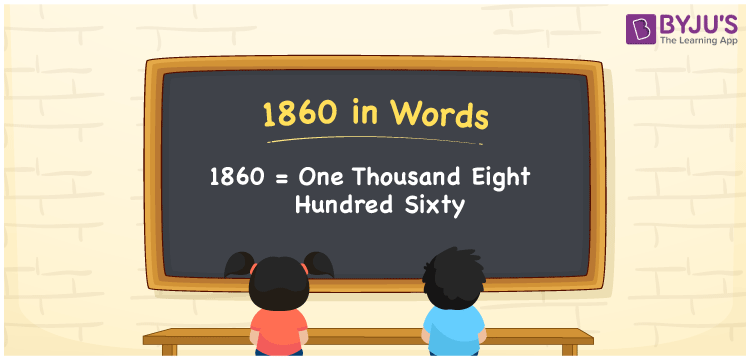# 1860 in Words

1860 in words is one thousand eight hundred sixty. If you purchased the books for Rs. 1860, then you can write it as “ I purchased the books for Rs. One thousand eight hundred sixty”. Also, the number 1860 has four digits and it is a cardinal number. In this article, let us discuss how to write the number 1860 in words using the place value system in detail.

 1860 in Words: One Thousand Eight Hundred Sixty. One Thousand Eight Hundred Sixty in Numerical Form: 1860.

## 1860 in English Words## How to Write 1860 in Words?

The following is the place value table for the number 1860:

 Thousands Hundreds Tens Ones 1 8 6 0

The expanded form of 1860 is as follows:

= 1 × Thousand + 8 × Hundred + 6 × Ten + 0 × One

= 1 × 1000 + 8 × 100 + 6 × 10 + 0 × 1

= 1000 + 800 + 60

= 1860

= One thousand eight hundred sixty

Hence, 1860 in words is one thousand eight hundred sixty.

1860 in words – One thousand eight hundred sixty

Is 1860 an odd number? – No

Is 1860 an even number? – Yes

Is 1860 a perfect square number? – No

Is 1860 a perfect cube number? – No

Is 1860 a prime number? – No

Is 1860 a composite number? – Yes

## Frequently Asked Questions on 1860 in Words

Q1

### How to write 1860 in words?

1860 in words is one thousand eight hundred sixty.

Q2

### Simplify 1800 + 60, and express it in words.

Simplifying 1800 + 60, we get 1860. Hence, 1860 in words is one thousand eight hundred sixty.

Q3

### convert one thousand eight hundred sixty into numbers.

One thousand eight hundred sixty in numbers is 1860.